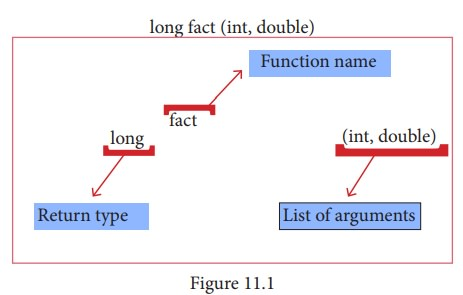Home | | Computer Science 11th std | User-defined Functions

# User-defined Functions

We can also define new functions to perform a specific task. These are called as user-defined functions. User-defined functions are created by the user.

User-defined Functions

## Introduction

We can also define new functions to perform a specific task. These are called as user-defined functions. User-defined functions are created by the user. A function can optionally define input parameters that enable callers to pass arguments into the function. A function can also optionally return a value as output. Functions are useful for encapsulating common operations in a single reusable block, ideally with a name that clearly describes what the function does.

## Function Definition

In C++, a function must be defined before it is used anywhere in the program. The general syntax of a function definition is:

Return_Data_Type Function_name(parameter list)

{

Body of the function

}

Note:

1. The Return_Data_Type is any valid data type of C++.

2. The Function_name is a user-defined identifier.

3. The parameter list, which is optional, is a list of parameters, i.e. a list of variables preceded by data types and separated by commas.

4. The body of the function comprises C++ statements that are required to perform the intended task of this function.

## Function Prototype

C++ program can contain any number of functions. But, it must always have only one main() function to begin the program execution. We can write the definitions of functions in any order as we wish. We can define the main() function first and all other functions after that or we can define all the needed functions prior to main(). Like a variable declaration, a function must be declared before it is used in the program. The declaration statement may be given outside the main() function.### The prototype above provides the following information to the compiler:

• The return value of the function is of type long.

• fact is the name of the function.

• the function is called with two arguments:

The first argument is of int data type.

The second argument is of double data type.

int display(int , int) // function prototype//

The above function prototype provides details about the return data type, name of the function and a list of formal parameters or arguments.

## Use of void command

void type has two important purposes:

• To indicate the function does not return a value

• To declare a generic pointer.

void data type indicates the compiler that the function does not return a value, or in a larger context void indicates that it holds nothing.

### For Example:

void fun(void)

The above function prototype tells compiler that the function fun() neither receives values from calling program nor return a value to the calling program.

## Accessing a function

The user-defined function should be called explicitly using its name and the required arguments to be passed. The compiler refers to the function prototype to check whether the function has been called correctly. If the argument type does not match exactly with the data type defined in the prototype, the compiler will perform type conversion, if possible. If type conversion is impossible, the compiler generates an error message.

### Example :

1. display() : calling the function without a return value and without any argument

2. display ( x, y) : calling the function without a return value and with arguments

3. x = display() : calling the function with a return value and without any argument

4. x = display (x, y) : calling the function with a return value and with arguments

### 1. Formal Parameters and Actual Parameters or Arguments

Arguments or parameters are the means to pass values from the calling function to the called function. The variables used in the function definition as parameters are known as formal parameters. The constants, variables or expressions used in the function call are known as actual parameters.### 2. Default arguments

In C++, one can assign default values to the formal parameters of a function prototype.

The Default arguments allows to omit some arguments when calling the function.

When calling a function,

• For any missing arguments, complier uses the values in default arguments for the called function.

• The default value is given in the form of variable initialization.

Example : void defaultvalue(int n1=10, n2=100);

• The default arguments facilitate the function call statement with partial or no arguments. Example : defaultvalue(x,y);

defaultvalue(200,150);

defaultvalue(150);

defaultvalue(x,150);

The default values can be included in the function prototype from right to left, i.e., we cannot have a default value for an argument in between the argument list.

Example :   void defaultvalue(int n1=10, n2);//invalid prototype

void defaultvalue(int n1, n2 = 10);//valid prototype

### 3. Constant Arguments

The constant variable can be declared using const keyword. The const keyword makes variable value stable. The constant variable should be initialized while declaring. The const modifier enables to assign an initial value to a variable that cannot be changed later inside the body of the function.

## Syntax :

<returntype><functionname> (const <datatype variable=value>)

Example:

• int minimum(const int a=10);

• float area(const float pi=3.14, int r=5);

### Program 11.16

#include <iostream>

using namespace std;

double area(const double r,const double pi=3.14)

{

return(pi*r*r);

}

int main ()

{

cout << "\nThe Area of Circle ="<<res;

return 0;

}

### Output:

The Area of Circle =78.5

If the variable value “r” is changed as r=25; inside the body of the function “area” then compiler will throw an error as “assignment of read-only parameter 'r'"

double area(const double r,const double pi=3.14)

{

r=25;

return(pi*r*r);

}

Tags : C++ | Computer Science , 11th Computer Science : Chapter 11 : Functions
Study Material, Lecturing Notes, Assignment, Reference, Wiki description explanation, brief detail
11th Computer Science : Chapter 11 : Functions : User-defined Functions | C++ | Computer Science